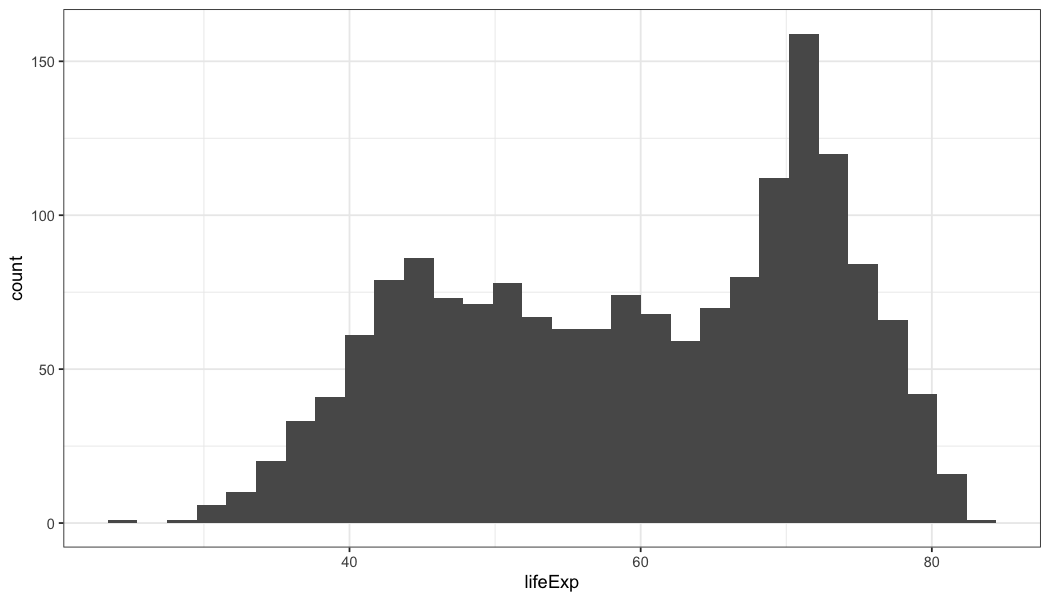``````#install.packages("tidyverse")
require(tidyverse) # The tidyverse package covered last time

# install.packages("ggthemes")
require(ggthemes) # for great visualization colors and themes.

# install.packages("maps")
require(maps) # for some maps data

# Gapminder data (for example)
#install.packages("gapminder")
require(gapminder)``````

# Data

The `Gapminder` dataset is a famous dataset used by Hans Rosling to visualize development outcomes. The data covers 1952 to 2007 in five year intervals and measures life expectancy, population, and GDP Per Capita.

``gapminder %>% head()``

# Get to know the data through visualization

Each of the following questions are targeted at making sure we understand our data better. A great way to get a “feel” for a dataset is to visualize it. Answer each of the below questions with a (publishable-quality) picture.

#### 1. How is `lifeExp`, `pop` and `gdpPercap` variables distributed?

``````# Life Expectancy
gapminder %>%
ggplot(aes(lifeExp)) +
geom_histogram(bins=30) +
theme_bw() ````````````
# Population
gapminder %>%
ggplot(aes(pop)) +
geom_histogram(bins=30) +
theme_bw() ``````